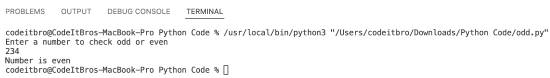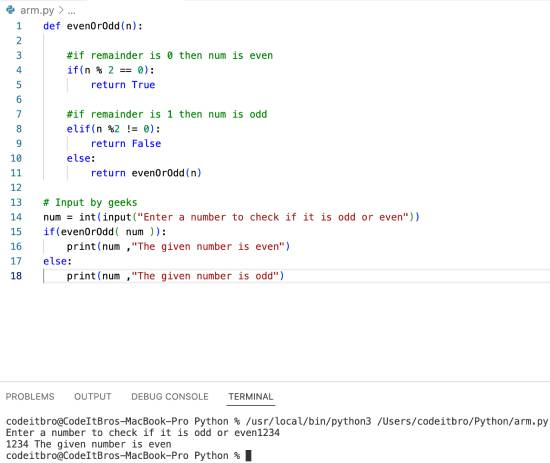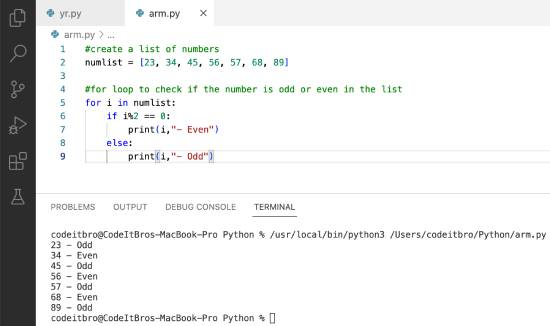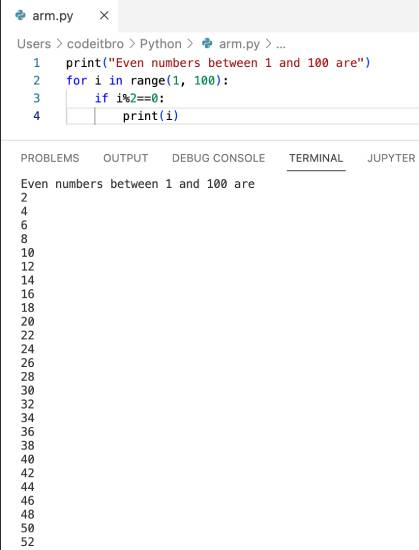# Python Program To Check If a Number is Odd or Even

103

In this simple Python program, you will learn to check if a number is odd or even. Before moving ahead, please ensure that you understand the basic concepts of Python programming, such as:

• Variables
• Python operators
• If-else statements

In case you have just started your Python journey, then here are some resources that you should check out:

## Python Program To Check If a Number is Odd or Even

Let’s now see how to check if a number is odd or even using Python code.

It is as simple as ABC. We will take input from the user and then check if the number is divisible by 2 or not. If the number is divisible by 2, it is an even number; otherwise, the given number is odd.

We will check the divisibility using the modulo operator (%) and use if-else Python statements to print if the number is odd or even.

Here’s the code for the same.

```#Python program to check if a number is odd or even

#Input number from user
number = int(input("Enter a number to check odd or even \n"))

#Checking if the nunmber is divisible by 2 or not

#If the number is divisible by 2 then it is an even number
if (number % 2) == 0:
print("Number is even")
#If the number is not divisble by 2 then it is an odd number
else:
print("Number is odd")```

Output:

Here’s the output of the above program.Other Python code examples you should check out:

## Python Program to Check Whether a Given Number is Even or Odd Recursively

Source Code:

```def evenOrOdd(n):

#if remainder is 0 then num is even
if(n % 2 == 0):
return True

#if remainder is 1 then num is odd
elif(n %2 != 0):
return False
else:
return evenOrOdd(n)

# Input by geeks
num = int(input("Enter a number to check if it is odd or even"))
if(evenOrOdd( num )):
print(num ,"The given number is even")
else:
print(num ,"The given number is odd")
```

Output:## Python Program To Check Even and Odd Numbers In a List

Source Code:

```#create a list of numbers
numlist = [23, 34, 45, 56, 57, 68, 89]

#for loop to check if the number is odd or even in the list
for i in numlist:
if i%2 == 0:
print(i,"- Even")
else:
print(i,"- Odd")```

Output:## Python Program to Print Even Numbers from 1 to 100 Using For Loop

Source Code:

```print("Even numbers between 1 and 100 are")
for i in range(1, 100):
if i%2==0:
print(i)```

Output:Here are some similar Python program examples you should check out:

## Python Program to Print Even Numbers from 1 to 100 Using While Loop

Source Code:

```print("Even numbers between 1 to 100 using while loop")
num = 2
while num <= 100:
print(num)
num += 2```

Output:

The output of this program will be similar to the output shown for the above Python example.

Must check out Python code snippets: